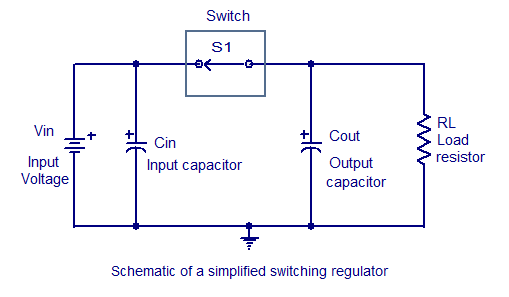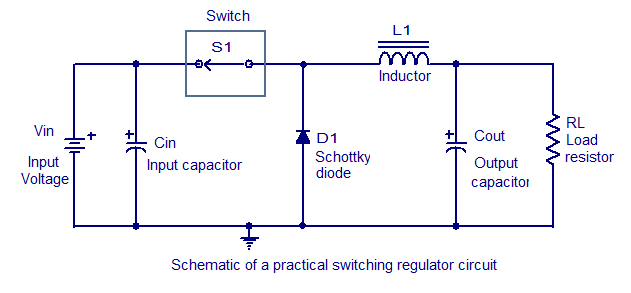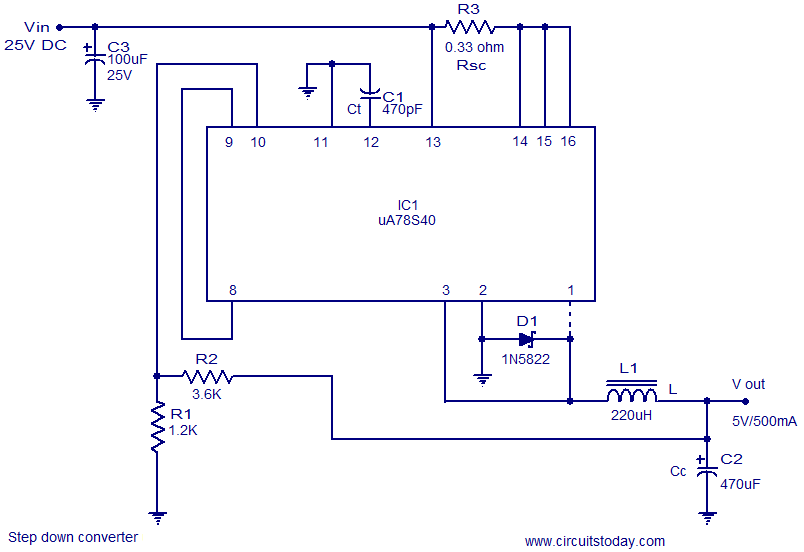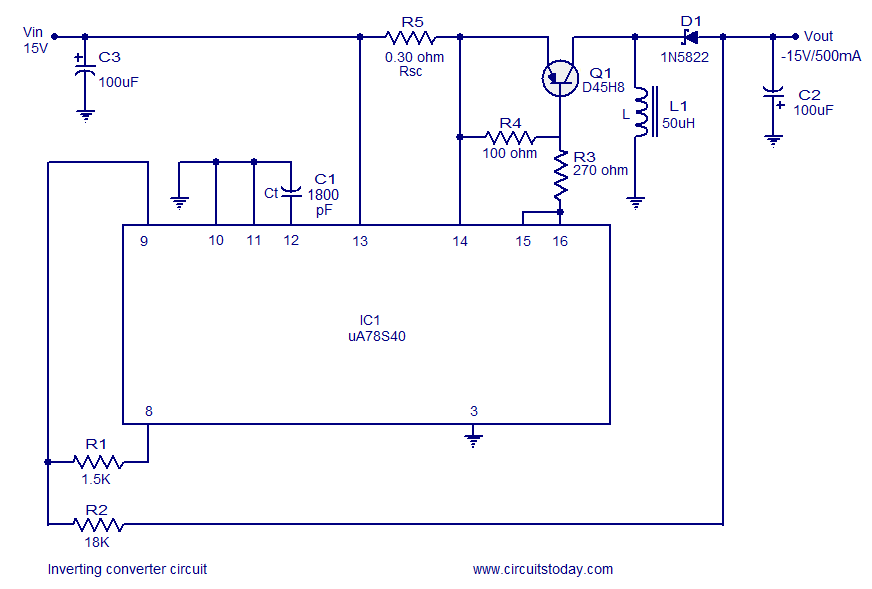Audio

[Audio][slideshow]

Switching regulator .

Switching regulators work by drawing small amounts of energy from the input source and transferring it step by step to the output. This task is attained by using an electronic switch (operating at a predetermined frequency) which works like a gate between the input energy source and the output. This gate controls the amount of charge that is transferred to the output load. The output voltage of the switching regulator depends on how much time the switch is maintained closed. If the OFF time of switch is long then less energy will be transferred to the output load and so the average output voltage will a be low. If the OFF time of switch is short then more energy will be transferred to the output load which results in a better average output voltage.The schematic of a basic switching regulator is shown below.Schematic of a simplified switching regulator circuit

When switch S1 is closed capacitor Cout is charged and when switch S1 is open the Cout discharges through the load. The duty cycle of the S1 determines how much energy is transferred to the output load. In simple words the capacitor Cout serves as a filter which converts the pulse waveform from the switch in to a steady voltage. The output voltage will be always a function of the input voltage and the duty cycle of the switch.Schematic of a practical switching regulator

The schematic of a practical switching regulator is shown above. This circuit has two additional components, a Schottky diode D1 and an inductor L1. These two components are present in almost all switching regulator circuits and they drastically improves the performance of the circuit. Let us see how the diode and inductor improves the performance of the regulator circuit.

When switch S1 is closed the inductor L1 opposes the rising current by creating an opposing electromagnetic field and this makes the diode D1 reverse biased and it behaves like an open switch. When switch S1 is made open the electromagnetic field that was induced in the inductor L1 will be discharging and this creates a current in the reverse polarity. This makes the diode D1 forward biased and it will remain in the conducting stage until the field in the inductor becomes zero. In simple words this action is similar to the charging and discharging of the output capacitor. Thus the combined effect of the inductor and diode improves the filtering capability of the output capacitor and so the circuit efficiency is improved.

uA78S40 based switching regulators.

Here are two switching voltage regulator circuits using uA78S40 IC from On Semiconductors. The first one is a step down converter while the second one is an inverting converter.

uA78S40 is a switching regulator IC that can be used for a variety of applications. The uA78S40 is an integrated switching regulator circuit which has built in circuitries for voltage reference with temperature compensation, oscillator with duty cycle control, high capacity switching element, an independent operational amplifier and independent diode. When voltages excess of 40V or output currents excess of 1.5A are required external switching transistors must be used. The features of uA78S40 include wide temperature range, adjustable output voltage (from 1.5V to 40V), peak output current of 1.5A, 80dB load regulation, 80dB line regulation, wide supply voltage range ( from 2.5V to 40V), very low standby current etc. The applications of this IC include step up converters, step down converters, inverting converters etc. The uA78S40 is available in a 16 pin DIP plastic package.

Step down converter circuit.Step down regulator using uA78S40

The circuit shown above is of a switching step down converter using uA78S40. The input voltage can be 25V DC and the output voltage is 5V @500mA. Ct is the timing capacitor for the internal oscillator while C3 is the input filter capacitor.C3 must be rated above 25V while C2 can be rated anything higher than 10V. The instantaneous output voltage (that is the voltage across output capacitor Cc) is fed back to the inverting input of the internal opamp using the resistor network comprising of R1 and R2. R2 and R1 can be used for setting the output voltage. Using the external rectifier diode D1 improves the overall efficiency of the circuit. If you need to use the internal diode of the IC instead of D1 then omit D1 and restore the track shown dotted.

Inverting converter circuit.Inverting converter circuit using uA78S40

An inverting converter is a circuit which reverses the polarity of a given input voltage. For example if 5V DC is applied at the input of an inverting converter, the output voltage will be -5V DC. A 15V inverting converter circuit using uA78S40 is shown above. Ct (in the circuit C1) is the timing capacitor for the ICs internal oscillator, C3 is the input filter capacitor and C2 is the output filter capacitor. Both C2 and C3 must be rated at least 25V. Resistors R1 and R2 forms a feedback network which feed backs a portion of the output voltage to the non inverting input of the Ics internal comparator. R2 and R1 can be used to set the output voltage. Transistor Q1 is the external switching transistor. The collector terminals of the internal driver and switching transistors are shorted and connected to the base of the external switching transistor through resistor R3.

Notes.

• uA78S40 must be mounted on a holder.
• Peak output current of uA78S40 is 1.5A.
• 1N5822 is a 3A Schottky diode. Do not replace it with an ordinary PN junction diode.
• Vout = 1.25 (1+ (R2/R1)) for step down converter.
• Vout = (1.25R2) / (R1) for inverting converter.

Switching Regulator Vs Linear regulator.

The controlling element for a linear regulator is an active device ( either a BJT or FET) operating inside its active region.In a linear regulator the difference between the input voltage and output voltage is dissipated as heat by the controlling element. This reduces the power efficiency. The controlling element requires a larger heatsink .

For a switching regulator the controlling element which is an electronic switch (a transistor or thyristor) has only two states, either ON (completely conducting) or OFF (completely open). This means that no power is wasted across the switching element and this result in better power efficiency. A well designed switching regulator can have up to 85% efficiency. The controlling element requires a smaller heatsink.

Few more switching regulator circuit that you may find interesting.

10V switching regulator using LM5007 : LM5007 is an integrated step down regulator IC which is very efficient and cost effective. This circuit produces 10V DC output from an input voltage range of 12 to 72V DC.

5V buck regulator using LM2678 : A buck regulator produces an output voltage which is lower than the input. The circuit is built around the Natinal Semicondcutors LM2678 IC. The input voltage range is from 8 to 40V and output is 5V DC.

Switching Regulator using LM1578 A : A simple switching regulator circuit designed using the LM1578A regulator IC from National semiconductors.The IC is very flexible and it can be operated in the buck, boost or inverting modes.

12V boost regulator circuit : A boost converter is a circuit which produces an output voltage which is higher than the input voltage. This circuit converts 5V DC to 12V DC. LM2698 is the heart of this circuit which is a boost converter IC from National Semiconductors.

[Lights][grids]